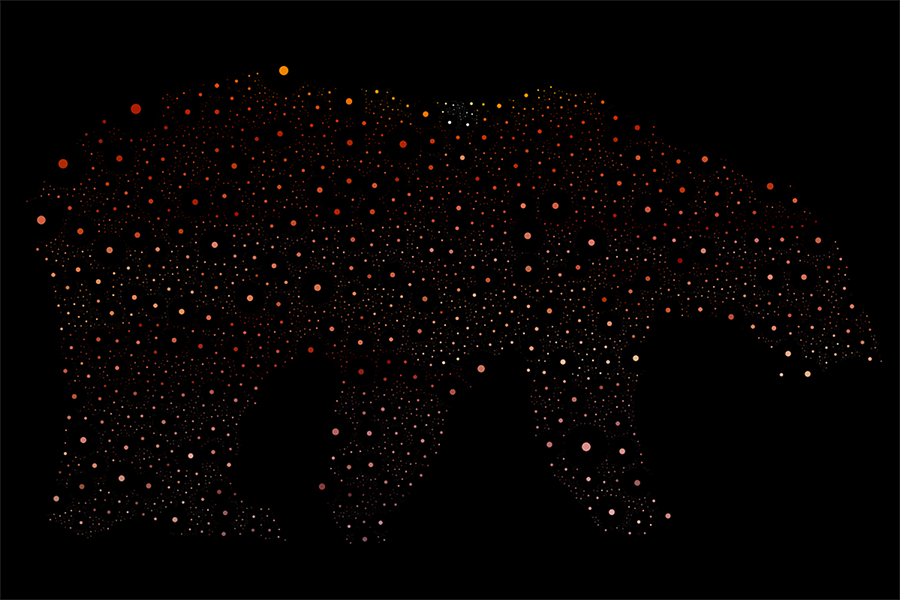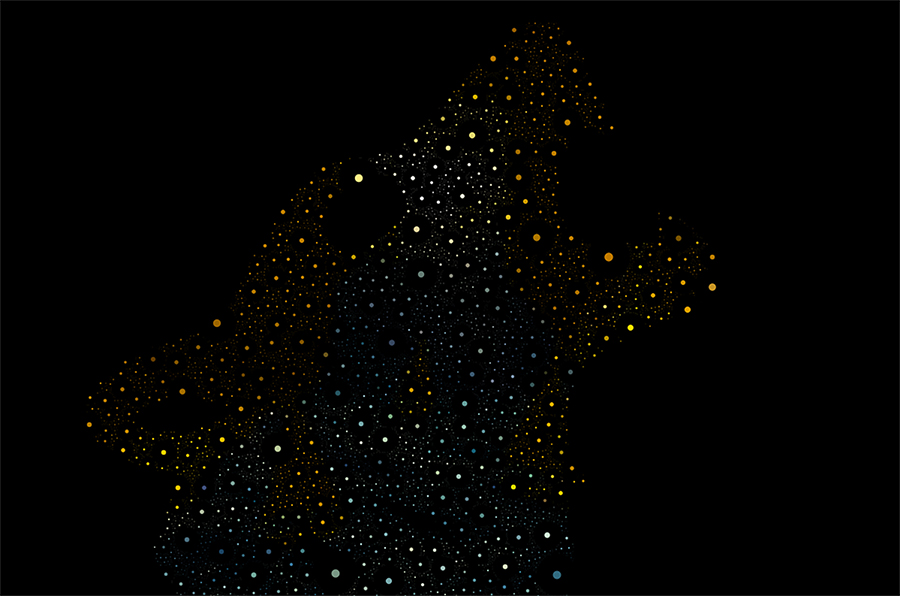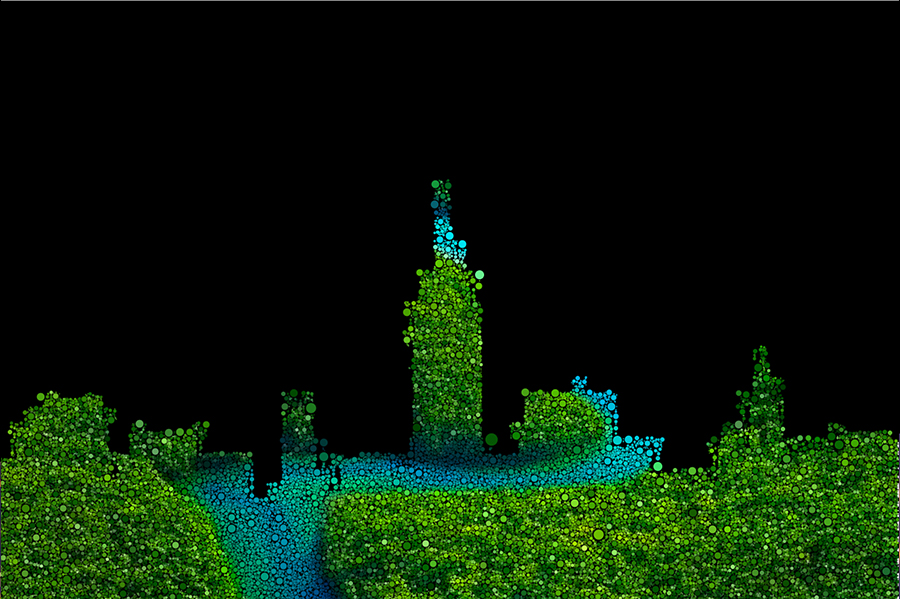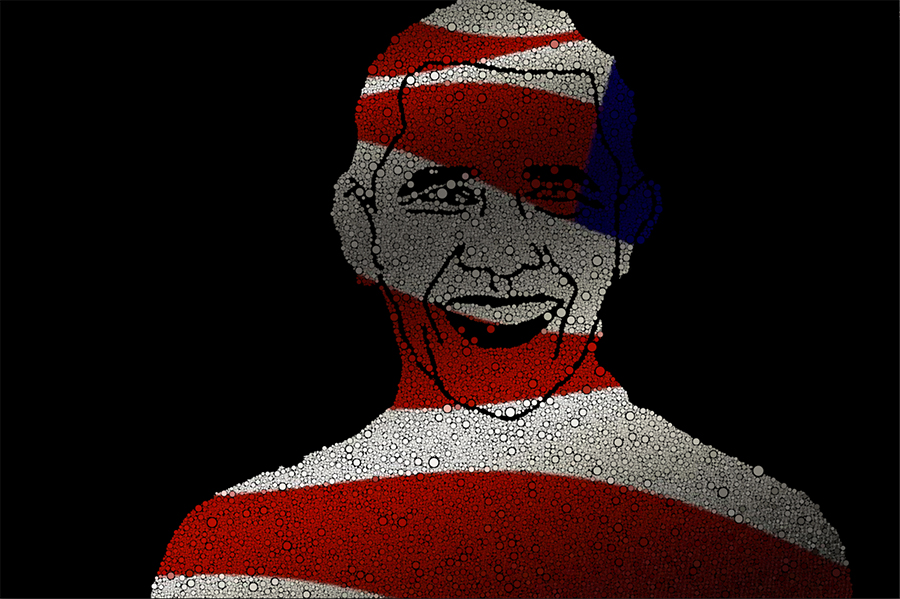# Circle packing in Processing

We’ll create a class called Circle

```class Circle { float x; float y; float r;```

```float growRate = 1; boolean growing = true;```

``` Circle(float x_, float y_){ x = x_; y = y_; r = 0.5; //r = 0.25; }```

` void grow(){`

```if (growing){ r = r+growRate; } }```

``` boolean edges(){ return(x+r > width || x-r height ||y-r < 0); }```

```void show(){ colrfromImg = clrImg.get(int(x),int(y));//store the color from the image noStroke(); fill(colrfromImg); ellipse(x,y,r/2.5,r/2.5); } };```

We’ll set up our sketch. There are two images being used. One defines the area where the circles will populate, the other image is used to extract a color palette and fill the circles with those colors.

```ArrayList circles; ArrayList spots;```

```PImage img; PImage clrImg; color colrfromImg;```
```void setup() { size(1200, 800); spots = new ArrayList (); img = loadImage("img42.jpg"); img.loadPixels(); clrImg = loadImage("img31.jpg"); clrImg.loadPixels(); for(int x =0; x<width; x++){ for(int y =0; y<height; y++){ int index = x + y * img.width; color c = img.pixels[index]; float b = brightness(c); if (b > 1){ spots.add(new PVector(x,y)); } } } circles = new ArrayList(); }```
```void draw() { background(0); int total =20; int count = 0; int attempts = 0; while (count < total) { Circle newC = newCircle(); if (newC !=null) { circles.add(newC); count++; } attempts ++; if (attempts > 5000) { noLoop();//stop completly the program //println("FINISHED"); break; } } for (Circle c : circles) { if (c.growing) { if (c.edges()) { c.growing = false; } else { //boolean overlapping = false; for (Circle other : circles) { if (c != other) { float d = dist(c.x, c.y, other.x, other.y); if (d < c.r + other.r) { c.growing = false; break; //overlapping = true; } } } } } c.show(); c.grow(); } }```
```Circle newCircle() { int r = int (random(0, spots.size())); PVector spot = spots.get(r); float x = spot.x; float y = spot.y; boolean valid = true; for (Circle c : circles) { float d = dist(x, y, c.x, c.y); //if (d < c.r + 2 ) if (d < c.r ) { valid = false; break; } } if (valid) { return new Circle (x, y); } else { return null; } };```